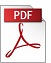### “Computational Performance Analysis of Nonlinear Dynamic Systems using Semi-infinite Programming”

Authors: Tor A. Johansen,
Affiliation: NTNU, Department of Engineering Cybernetics
Reference: 2001, Vol 22, No 1, pp. 15-27.

Keywords: Hamilton-Jacobi inequality, stability, Lyapunov functions, convex optimization, performance

Abstract: For nonlinear systems that satisfy certain regularity conditions it is shown that upper and lower bounds on the performance (cost function) can be computed using linear or quadratic programming. The performance conditions derived from Hamilton-Jacobi inequalities are formulated as linear inequalities defined pointwise by discretizing the state-space when assuming a linearly parameterized class of functions representing the candidate performance bounds. Uncertainty with respect to some system parameters can be incorporated by also gridding the parameter set. In addition to performance analysis, the method can also be used to compute Lyapunov functions that guarantees uniform exponential stability.PDF (1590 Kb)        DOI: 10.4173/mic.2001.1.2

References:
 JOHANSEN, T.A. (2000). Computation of Lyapunov Functions for Smooth Nonlinear Systems Using Convex Optimization, Automatica, Vol. 36.
 RANTZER, A. (1999). Dynamic Programming Via Convex Optimization, In: Preprints IFAC World Congress, Beijing, Vol. D, pp. 491-496.
 JOHANSSON, M. RANTZER, A. (1998). Computation of Piecewise Quadratic Lyapunov Functions for Hybrid Systems, IEEE Trans. Automatic. Control, Vol. 43, pp. 555-559 doi:10.1109/9.664157
 RANTZER, A. JOHANSSON, M. (2000). Piecewise Linear Quadratic Optimal Control, IEEE Trans. Automatic Control, Vol. 45, pp. 629-637.
 PETTERSON, S. LENNARTSON, B. (1997). Exponential Stability Analysis of Nonlinear Systems Using LMIs, In: Proc IEEE Conf Decision and Control, San Diego.
 JOHANSEN, T.A. (1999). Characterization of Lyapunov Functions for Smooth Nonlinear Systems Using LMIs, In: Preprints IFAC World Congress, Beijing, Vol. 3, pp. 387-392.
 OHTA, Y. ONISHI, M. (1999). Stability Analysis by Using Piecewise Linear Lyapunov Functions, In: Preprints IFAC World Congress, Beijing, Vol. D, pp. 515-520.
 JULIAN, P., GUIVANT, J. DESAGES, A. (1999). A Parameterization of Piecewise Linear Lyapunov Functions via Linear Programming, Int. J. Control, Vol. 72, pp. 702-715 doi:10.1080/002071799220876
 BRANICKY, M.D. (1995). Studies in Hybrid Systems: Modeling, Analysis and Control, PhD Thesis, MIT, Laboratory for Information and Decision Systems.
 HEDLUND, S. RANTZER, A. (1999). Optimal Control of Hybrid Systems, In: Proc. IEEE Conference on Decision and Control, Phoenix, AZ.
 LIN, W. BYRNES, C.I. (1995). Zero-state Observability and Stability of Discrete-time Nonlinear Systems, Automatica, Vol. 31, pp. 269-274 doi:10.1016/0005-1098(94)00088-Z
 KHALIL, H.K. (1992). Nonlinear Systems, Macmillan, New York.
 TANAKA, Y., FUKUSHIMA, M. IBARAKI, T. (1988). A Comparative Study of Several Semi-infinite Nonlinear Programming Algorithms, European Journal of Operational Research, Vol. 36, pp. 92-100 doi:10.1016/0377-2217(88)90010-0
 POLAK, E. (1997). Optimization: Algorithms and Consistent Approximation, Springer, New York.
 JOHANSEN, T.A., PETERSEN, I. SLUPPHAUG, O. (2000). Explicit Suboptimal Linear Quadratic Regulation with Input and State Constraints, Proc. IEEE CDC, Sydney.

BibTeX:
@article{MIC-2001-1-2,
title={{Computational Performance Analysis of Nonlinear Dynamic Systems using Semi-infinite Programming}},
author={Johansen, Tor A.},
journal={Modeling, Identification and Control},
volume={22},
number={1},
pages={15--27},
year={2001},
doi={10.4173/mic.2001.1.2},
publisher={Norwegian Society of Automatic Control}
};